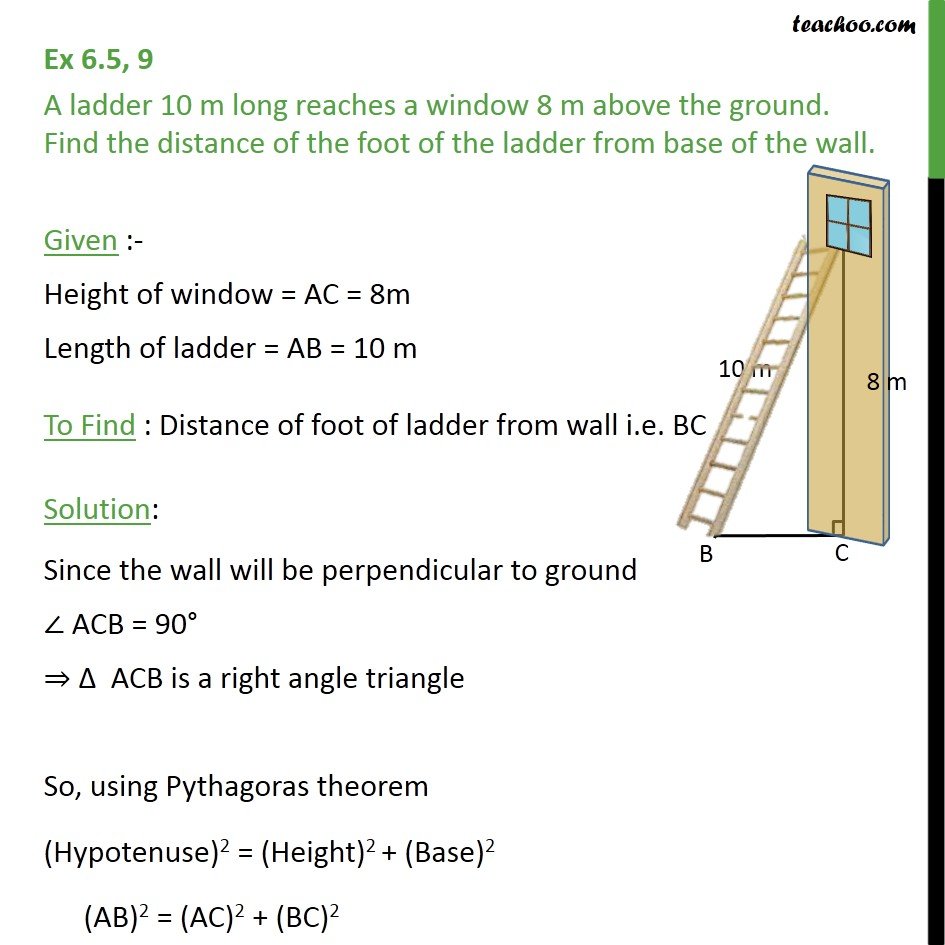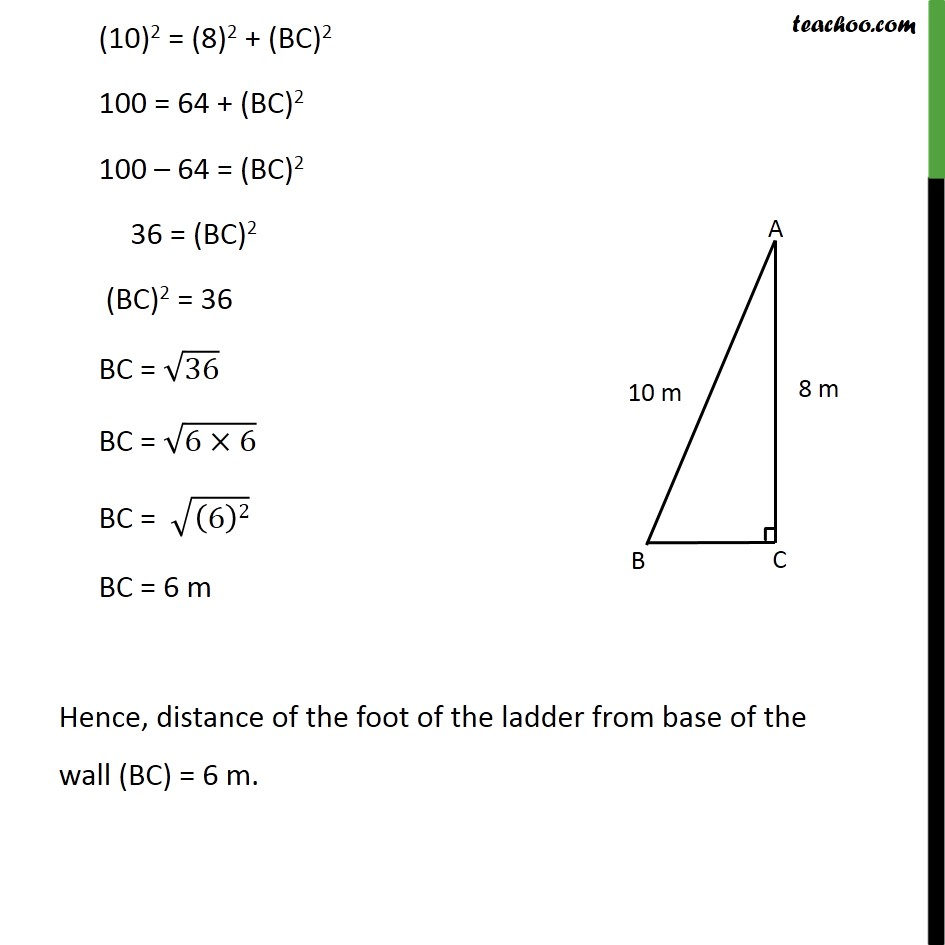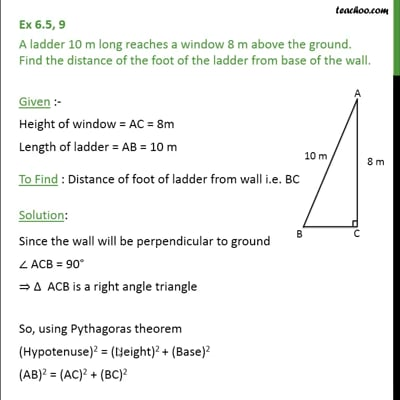Ex 6.5

Chapter 6 Class 10 Triangles
Serial order wiseThis video is only available for Teachoo black users

Solve all your doubts with Teachoo Black (new monthly pack available now!)

### Transcript

Ex 6.5, 9 A ladder 10 m long reaches a window 8 m above the ground. Find the distance of the foot of the ladder from base of the wall. Given :- Height of window = AC = 8m Length of ladder = AB = 10 m To Find : Distance of foot of ladder from wall i.e. BC Solution: Since the wall will be perpendicular to ground ACB = 90 ACB is a right angle triangle So, using Pythagoras theorem (Hypotenuse)2 = (Height)2 + (Base)2 (AB)2 = (AC)2 + (BC)2 (10)2 = (8)2 + (BC)2 100 = 64 + (BC)2 100 64 = (BC)2 36 = (BC)2 (BC)2 = 36 BC = 36 BC = (6 6) BC = ((6)2) BC = 6 m Hence, distance of the foot of the ladder from base of the wall (BC) = 6 m.